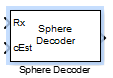# Sphere Decoder

Decode input using a sphere decoder

## Library

MIMO

•## Description

This block decodes the symbols sent over `Nt` antennas using the sphere decoding algorithm.

### Data Type

For information about the data types each block port supports, see the Supported Data Type table on this page. The output signal inherits the data type from the inputs.

### Algorithm

This block implements the algorithm, inputs, and outputs described on the `comm.SphereDecoder` System object™ block reference page. The object properties correspond to the block parameters.

## Parameters

Signal constellation

Specify the number of points in the signal constellation to which the bits are mapped. This value must be a complex column vector. The length of the vector must be a power of two. The block uses the same constellation for each transmit antenna. The default setting is a QPSK constellation with an average power of 1.

Bit mapping per constellation point

Specify the bit mapping that the block uses for each constellation point. This value must be a numerical matrix. he matrix size must be [ConstellationLength bitsPerSymbol], where ConstellationLength represents the length of the Signal constellation parameter value and bitsPerSymbol represents the number of bits that each symbol encodes. The default matrix size is [0 0; 0 1; 1 0; 1 1], which matches the default value of the Signal constellation property.

Specify the initial search radius for the decoding algorithm as `Infinity` or `ZF solution`.

When you select `Infinity`, the block sets the initial search radius to Inf. When you select `ZF solution`, the block 'sets the initial search radius to the zero-forcing solution. The zero-forcing solution is calculated by the pseudo-inverse of the input channel when decoding. Large constellations and/or antenna counts can benefit from the initial reduction in the search radius. In most cases, however, the extra computation of the `ZF Solution` will not provide a benefit.

Decision method

Specify the decoding decision method as `Soft` or `Hard`. When you select `Soft` the block outputs log-likelihood ratios (LLRs), or soft bits. When you select set to `Hard`, the block converts the soft LLRs to bits. The hard decision output logical array follows the mapping of a `0` for a negative LLR and `1` for all other values.

Simulation using

Specify if the block simulates using `Code generation` or `Interpreted execution`. The default is ```Interpreted execution```.

## Supported Data Type

PortSupported Data Types
Rx
• Double-precision floating point

cEst
• Double-precision floating point

Output
• Double-precision floating point

• Boolean (Hard-decision method)

## Limitations

• The output LLR values are not scaled by the noise variance. For coded links employing iterative coding (LDPC or turbo) or MIMO OFDM with Viterbi decoding, the output LLR values should be scaled by the channel state information to achieve better performance.

## Algorithms

This block implements the algorithm, inputs, and outputs described on the Sphere Decoder System object reference page. The object properties correspond to the block parameters.

## Extended Capabilities

### Objects

Introduced in R2013b